GeeksforGeeks App
Open AppBrowser
Continue

# How to verify Pyspark dataframe column type ?

While working with a big Dataframe, Dataframe consists of any number of columns that are having different datatypes. For pre-processing the data to apply operations on it, we have to know the dimensions of the Dataframe and datatypes of the columns which are present in the Dataframe.

In this article, we are going to know how to verify the column type of the Dataframe. For verifying the column type we are using dtypes function. The dtypes function is used to return the list of tuples that contain the Name of the column and column type.

Syntax: df.dtypes()

where, df is the Dataframe

At first, we will create a dataframe and then see some examples and implementation.

## Python

 `# importing necessary libraries``from` `pyspark.sql ``import` `SparkSession` `# function to create new SparkSession``def` `create_session():``  ``spk ``=` `SparkSession.builder \``      ``.master(``"local"``) \``      ``.appName(``"Product_details.com"``) \``      ``.getOrCreate()``  ``return` `spk` `def` `create_df(spark,data,schema):``  ``df1 ``=` `spark.createDataFrame(data,schema)``  ``return` `df1` `if` `__name__ ``=``=` `"__main__"``:` `  ``# calling function to create SparkSession``  ``spark ``=` `create_session()``    ` `  ``input_data ``=` `[(``"Mobile"``,``112345``,``4.0``,``12499``),``          ``(``"LED TV"``,``114567``,``4.2``,``49999``),``          ``(``"Refrigerator"``,``123543``,``4.4``,``13899``),``          ``(``"Washing Machine"``,``113465``,``3.9``,``6999``),``          ``(``"T-shirt"``,``124378``,``4.1``,``1999``),``          ``(``"Jeans"``,``126754``,``3.7``,``3999``),``          ``(``"Running Shoes"``,``134565``,``4.7``,``1499``),``          ``(``"Face Mask"``,``145234``,``4.6``,``999``)]` `  ``schema ``=` `[``"Name"``,``"ID"``,``"Rating"``,``"Price"``]``  ` `  ``# calling function to create dataframe``  ``df ``=` `create_df(spark,input_data,schema)` `  ``# visualizing the dataframe``  ``df.show()`

Output: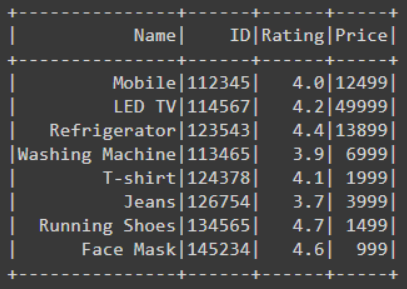Example 1: Verify the column type of the Dataframe using dtypes() function

In the below example code, we have created the Dataframe then for getting the column types of all the columns present in the Dataframe we have used dtypes function by writing df.dtypes using with f string while finding the datatypes of all the columns we have printed also. This gives a list of tuples that contains the name and datatype of the columns.

## Python

 `# finding data type of the all the``# column using dtype function and``# printing``print``(f``'Data types of all the columns is : {df.dtypes}'``)` `# visualizing the dataframe``df.show()`

Output: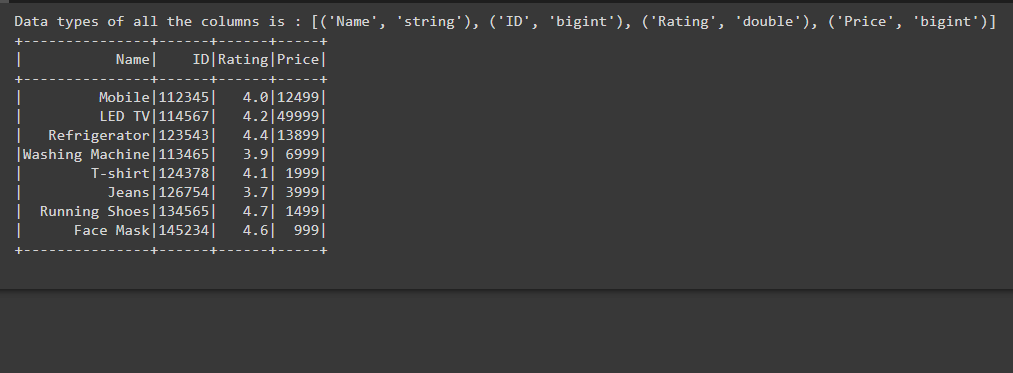Example 2: Verify the specific column datatype of the Dataframe

In the below code after creating the Dataframe we are finding the Datatype of the particular column using dtypes() function by writing dict(df.dtypes)[‘Rating’], here we are using dict because as we see in the above example df.dtypes return the list of tuples that contains the name and datatype of the column. So using dict we are typecasting tuple into the dictionary.

As we know in the dictionary the data is stored in key and value pair, while writing dict(df.dtypes)[‘Rating’] we are giving the key i.e, ‘Rating’ and extracting its value of that is double, which is the datatype of the column. So in this way, we can find out the datatype of column type while passing the specific name of the column.

## Python

 `# finding data type of the Rating``# column using dtype function``data_type ``=` `dict``(df.dtypes)[``'Rating'``]` `# printing``print``(f``'Data type of Rating is : {data_type}'``)` `# visualizing the dataframe``df.show()`

Output: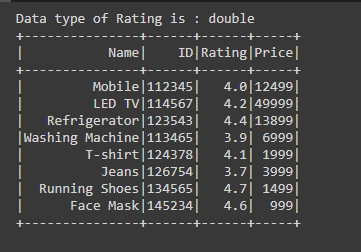Example 3: Verify the column type of the Dataframe using for loop

After creating the Dataframe, for finding the datatypes of the column with column name we are using df.dtypes which gives us the list of tuples.

While iterating we are getting the column name and column type as a tuple then printing the name of the column and column type using print(col,”,”,col). In this way, we are getting every column name and column type using by iterating.

## Python

 `print``(``"Datatype of the columns with column names are:"``)` `# finding datatype of all column with``# column name using for loop``for` `col ``in` `df.dtypes:``  ` `  ``# printing the column and datatype``  ``# of that column``  ``print``(col[``0``],``","``,col[``1``])` `# visualizing the dataframe``df.show()`

Output: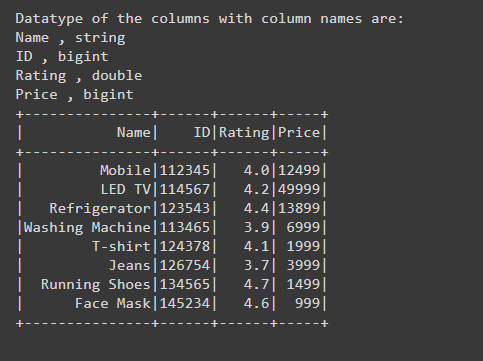Example 4: Verify the column type of the Dataframe using schema

After creating the Dataframe for verifying the column type we are using printSchema() function by writing df.printSchema() through this function schema of the Dataframe is printed which contains the datatype of each and every column present in Dataframe. So, using printSchema() function also we can easily verify the column type of the PySpark Dataframe.

## Python

 `# printing the schema of the Dataframe``# using printschema function``df.printSchema()` `# visualizing the dataframe``df.show()`

Output: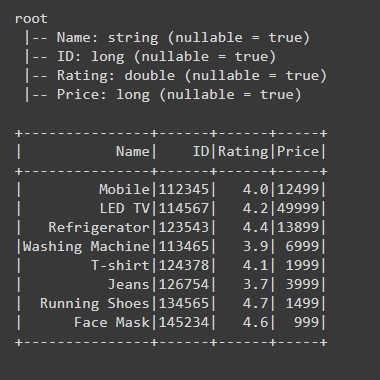My Personal Notes arrow_drop_up